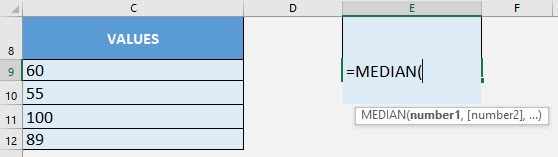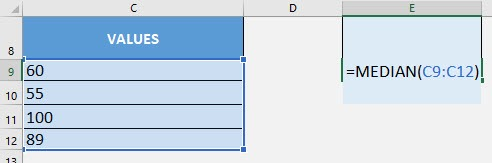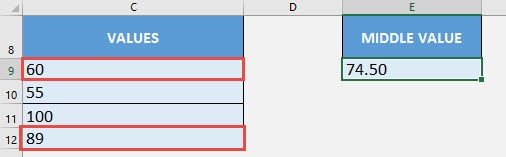What does it do?

Gets the middle number in the set of numbers

Formula breakdown:

=MEDIAN(number1, [number2], …)

What it means:

=MEDIAN(first number, [succeeding numbers in the set], …)

You have a list of values and you want to get the median or middle of those values. Excel’s MEDIAN Formula can easily get that for you!

An important think to note though, if it’s an odd number of values, it will simply get the middle value. But if it’s an even number of values, it will get the 2 middle values, then get their average!

I explain how you can do this below:STEP 1: We need to enter the MEDIAN function in a blank cell:

## =MEDIAN(STEP 2: The MEDIAN arguments:

## number1, [number2], …

What is the range of values?

Select the cells containing your values:

## =MEDIAN(C9:C12)You now have your median! It is calculated as the average of the 2 middle values since we have an even number of values: (60 + 89) / 2 = 74.50How to Use the MEDIAN Formula in Excel